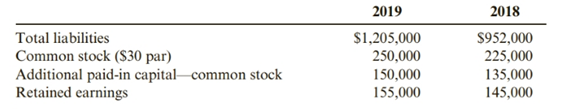Chapter 12, Problem 50CE### Cornerstones of Financial Accounti...

4th Edition
Jay Rich + 1 other
ISBN: 9781337690881

#### Solutions

Chapter
Section### Cornerstones of Financial Accounti...

4th Edition
Jay Rich + 1 other
ISBN: 9781337690881
Textbook Problem
3 views

# Debt Management RatiosSelected data from the financial statements of Lopez Company follow.Required:Determine whether the debt to equity ratio is increasing or decreasing and whether Lopez should be concerned.

To determine

Introduction:

Debt-equity ratio is calculated by dividing the total debt of the company to the shareholders fund. It is used to compute the financial leverage of a company. it describes the company’s structure which is divided into debt and equity.

To find:

The debt equity ratio of Lopez company for 2018 and 2019 and comparing the results.

Explanation

Debt-equity ratio=Total liabilitiesTotal equity

Total equity for 2018 = Common stock + additional paid-in-capital + Retained earnings

= $225,000 +$135,000 + $145,000 =$505,000

Total equity for 2019 = Common stock + additional paid-in-capital + Retained earni

### Still sussing out bartleby?

Check out a sample textbook solution.

See a sample solution

#### The Solution to Your Study Problems

Bartleby provides explanations to thousands of textbook problems written by our experts, many with advanced degrees!

Get Started

#### Find more solutions based on key concepts